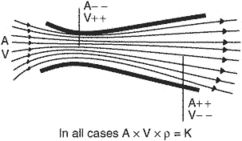# equation of continuity

## equation of continuity

[i′kwā·zhən əv ‚känt·ən′ü·əd·ē]
(physics)
McGraw-Hill Dictionary of Scientific & Technical Terms, 6E, Copyright © 2003 by The McGraw-Hill Companies, Inc.

## equation of continuityThe equation of continuity states that (a) air mass flow is constant, as mass can neither be created nor destroyed, and (b) the product of a cross-sectional area (A), velocity (V), and density (ρ) is constant (K), or AVρ = K. It assumes that because pressure changes are small, they have little effect on density and, therefore, AV= K or V= K/A. Hence, a reduced cross section gives a greater velocity.
References in periodicals archive ?
Determining viscous dissipation requires the velocity field to be calculated first by solving the equation of continuity (Eq.
Despite the presence of [rho]V, the equation of continuity (2.6) still holds and ensures that, apart from the boundaries, there are no forcing current terms inside the capacitor.
Assuming the vortex seepage flow and distribution of the resistance element evenly over the section we will use the generalized Darcy law and equation of continuity. We assume that the compressibility of the skeleton and pore fluid is low, which l eads to a linear dependence of the porosity of the soil pressure.
The equation of continuity for the refrigerant, the equation of pressure loss, and the equation of energy are as follows:
According to the above assumptions, the blood flow dynamics is governed by the equation of continuity
that, written in the form of an equation of continuity, leads to the following expression for the velocity of propagation of energy (ray velocity):
The equation of continuity (1) is identically satisfied if we take the stream function [psi](x, y) such that
But equation of continuity for steady and ideal flow is From equations (1) and (2), we get .
The equations for the flow inside the drops are given by the equation of continuity for an incompressible fluid and the Navier-Stokes equation within the quasistatic approximation, so that
In view of the equation of continuity we introduce the stream function [psi] as

Site: Follow: Share:
Open / Close